## How to Calculate and Solve for Volume of Superheated Steam | Enthalpy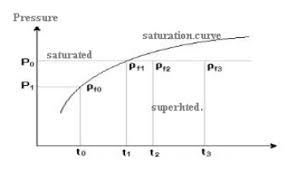The volume of superheated steam is illustrated by the image above.

To compute for volume of superheated steam, three essential parameters are needed and these parameters are Specific Volume of Dry Steam (ug), Superheated Temprature (tsup) and Saturated Temprature (ts).

The formula for calculating the volume of superheated steam:

vsup = ugTsup / Ts

Where:

vsup = Volume of Superheated Steam
ug = Specific Volume of Dry Steam
Tsup = Superheated Temprature
Ts = Saturated Temprature

Let’s solve an example;
Find the volume of superheated steam when the specific volume of dry steam is 32, the superheated temperature is 24 and the saturated temperature is 14.

This implies that;

ug = Specific Volume of Dry Steam = 32
Tsup = Superheated Temprature = 24
Ts = Saturated Temprature = 14

vsup = ugTsup / Ts
vsup = 32 x 24 / 14
vsup = 768 / 14
vsup = 54.85

Therefore, the volume of superheated steam is 54.85 m3.

Calculating the Specific Volume of Dry Steam when the Volume of Superheated Steam, the Superheated Temperature and the Saturated Temperature is Given.

ug = vsup x Ts / Tsup

Where:

ug = Specific Volume of Dry Steam
vsup = Volume of Superheated Steam
Tsup = Superheated Temprature
Ts = Saturated Temprature

Let’s solve an example;
Find the specific volume of dry steam when the volume of superheated steam is 10, the superheated temperature is 4 and the saturated temperature is 8.

This implies that;

vsup = Volume of Superheated Steam = 10
Tsup = Superheated Temprature = 4
Ts = Saturated Temprature = 8

ug = vsup x Ts / Tsup
ug = 10 x 8 / 4
ug = 80 / 4
ug = 20

Therefore, the specific volume of dry steam is 20 m3.

## How to Calculate and Solve for Volume of Wet and Dry Steam | Enthalpy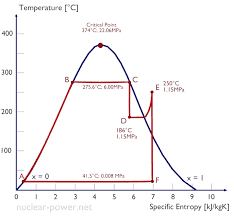The volume of wet and dry steam is illustrated by the image above.

To compute for the volume of wet and dry steam, three essential parameters are needed and these parameters are Dryness Fraction (x), Specific Volume of Dry Steam (ug) and Specific Volume of Water (uf).

The formula for calculating volume of wet and dry steam:

v = xug + (1 – x)uf

Where:

v = Volume of Wet and Dry Steam
x = Dryness Fraction
ug = Specific Volume of Dry Steam
uf = Specific Volume of Water

Let’s solve an example;
Given that the dryness fraction is 22, the specific volume of dry steam is 12 and the specific volume of water is 8. Find the volume of wet and dry steam?

This implies that;

x = Dryness Fraction = 22
ug = Specific Volume of Dry Steam = 12
uf = Specific Volume of Water = 8

v = xug + (1 – x)uf
v = 22 x 12 + (1 – 22) x 8
v = 22 x 12 + (-21) x 8
v = 264 + -168
v = 96

Therefore, the volume of wet and dry steam is 96 m3.

## How to Calculate and Solve for Enthalpy of Superheated Steam | Enthalpy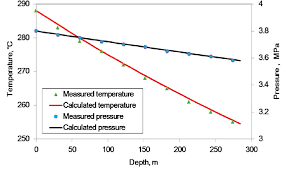The image above represents enthalpy of superheated steam.

To compute for enthalpy of superheated steam, five essential parameters are needed and these parameters are Constant Pressure (Cps), Enthalpy of Water (hf), Enthalpy of Vapourization (hfg), Superheated Temprature (Tsup) and Saturated Temprature (Ts).

The formula for calculating enthalpy of superheated steam:

hsup = hf + hfg + Cps(Tsup – Ts)

Where:

hsup = Enthalpy of Superheated Steam
Cps = Constant Pressure
hf = Enthalpy of Water
hfg = Enthalpy of Vapourization
Tsup = Superheated Temprature
Ts = Saturated Temprature

Let’s solve an example;
Given that the constant pressure is 2, the enthalpy of water is 4, the enthalpy of vapourization is 6, the superheated temperature is 8 and the saturated temperatue is 10. Find the enthalpy of superheated steam?

This implies that;

Cps = Constant Pressure = 2
hf = Enthalpy of Water = 4
hfg = Enthalpy of Vapourization = 6
Tsup = Superheated Temprature = 8
Ts = Saturated Temprature = 10

hsup = hf + hfg + Cps(Tsup – Ts)
hsup = 4 + 6 + 2 x (8 – 10)
hsup = 10 + 2 x (-2)
hsup = 10 + (-4)
hsup = 6

Therefore, the enthalpy of superheated steam is 6 J/Kg.

Calculating the Constant Pressure when the Enthalpy of Superheatred Steam, the Enthalpy of Water, the Enthalpy of Vapourization, the Superheated Temperature and the Saturated Temperature is Given.

Cps = hsup – hf – hfg / Tsup + Ts

Where:

Cps = Constant Pressure
hsup = Enthalpy of Superheated Steam
hf = Enthalpy of Water
hfg = Enthalpy of Vapourization
Tsup = Superheated Temprature
Ts = Saturated Temprature

Let’s solve an example;
Find the Constant Pressure when the enthalpy of superheated steam is 30, the enthalpy of water is 10, the enthalpy of vapourization is 4, the superheated temperature is 8 and the saturated temperature is 2.

This implies that;

hsup = Enthalpy of Superheated Steam = 30
hf = Enthalpy of Water = 10
hfg = Enthalpy of Vapourization = 4
Tsup = Superheated Temprature = 8
Ts = Saturated Temprature = 2

Cps = hsup – hf – hfg / Tsup + Ts
Cps = 30 – 10 – 4 / 8 + 2
Cps = 16 / 10
Cps = 1.6

Therefore, the constant pressure is 1.6.

## How to Calculate and Solve for Enthalpy of Wet Stream | Enthalpy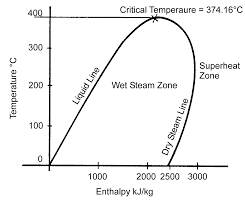The image above represents enthalpy for wet steam.

To compute for enthalpy for wet steam, three essential parameters are needed and these parameters are Dryness Fraction (x), Enthalpy of Water (hf) and Enthalpy of Vapourization (hfg).

The formula for calculating enthalpy for wet steam:

h = hf + xhfg

Where:

h = Enthalpy of Wet Steam
x = Dryness Fraction
hf = Enthalpy of Water
hfg = Enthalpy of Vapourization

Let’s solve an example;
Find the enthalpy of wet steam when the dryness fraction is 4, the enthalpy of water is 6 and the enthalpy of vapourization is 8.

This implies that;

x = Dryness Fraction = 4
hf = Enthalpy of Water = 6
hfg = Enthalpy of Vapourization = 8

h = hf + xhfg
h = 6 + 4 x 8
h = 6 + 32
h = 38

Therefore, the Enthalpy of Wet Steam is 38 J/Kg.

Calculating the Dryness Fraction when the Enthalpy of Wet Steam, the Enthalpy of Water and the Enthalpy of Vapourization is Given.

x = h – hf / hfg

Where;

x = Dryness Fraction
h = Enthalpy of Wet Steam
hf = Enthalpy of Water
hfg = Enthalpy of Vapourization

Let’s solve an example;
Find the dryness fraction when the enthalpy of wet steam is 14, the enthalpy of water is 8 and the enthalpy of vapourization is 2.

This implies that;

h = Enthalpy of Wet Steam = 14
hf = Enthalpy of Water = 8
hfg = Enthalpy of Vapourization = 2

x = h – hf / hfg
x = 14 – 8 / 2
x = 6 / 2
x = 3

Therefore, the dryness fraction is 3.

## How to Calculate and Solve for Dryness Fraction | Enthalpy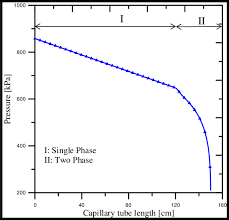The image above represents the dryness fraction.

To compute for dryness fraction, two essential parameters are needed and these parameters are Mass of Dry Steam Contained in Steam Considered (ms) and Weight of Water Particles in Suspension in Steam Considered (mw).

The formula for calculating dryness fraction:

x = ms ms + mw

Where:

x = Dryness Fraction
ms = Mass of Dry Steam Contained in Steam Considered
mw = Weight of Water Particles in Suspension in Steam Considered

Let’s solve an example;
Find the dryness fraction when the mass of dry steam contained in steam considered is 21 and the weigth of water particles in suspension in steam considered is 10.

This implies that;

ms = Mass of Dry Steam Contained in Steam Considered = 21
mw = Weight of Water Particles in Suspension in Steam Considered = 10

x = msms + mw
x = 21 / 21 + 10
x = 21 / 31
x = 0.677

Therefore, the dryness fraction is 0.677.